SSC CGL Previous Year Questions: Profit & Loss - 3

# SSC CGL Previous Year Questions: Profit & Loss - 3 - SSC CGL

Test Description

## 40 Questions MCQ Test SSC CGL (Tier - 1) - Previous Year Papers (Topic Wise) - SSC CGL Previous Year Questions: Profit & Loss - 3

SSC CGL Previous Year Questions: Profit & Loss - 3 for SSC CGL 2023 is part of SSC CGL (Tier - 1) - Previous Year Papers (Topic Wise) preparation. The SSC CGL Previous Year Questions: Profit & Loss - 3 questions and answers have been prepared according to the SSC CGL exam syllabus.The SSC CGL Previous Year Questions: Profit & Loss - 3 MCQs are made for SSC CGL 2023 Exam. Find important definitions, questions, notes, meanings, examples, exercises, MCQs and online tests for SSC CGL Previous Year Questions: Profit & Loss - 3 below.
Solutions of SSC CGL Previous Year Questions: Profit & Loss - 3 questions in English are available as part of our SSC CGL (Tier - 1) - Previous Year Papers (Topic Wise) for SSC CGL & SSC CGL Previous Year Questions: Profit & Loss - 3 solutions in Hindi for SSC CGL (Tier - 1) - Previous Year Papers (Topic Wise) course. Download more important topics, notes, lectures and mock test series for SSC CGL Exam by signing up for free. Attempt SSC CGL Previous Year Questions: Profit & Loss - 3 | 40 questions in 40 minutes | Mock test for SSC CGL preparation | Free important questions MCQ to study SSC CGL (Tier - 1) - Previous Year Papers (Topic Wise) for SSC CGL Exam | Download free PDF with solutions
 1 Crore+ students have signed up on EduRev. Have you?
SSC CGL Previous Year Questions: Profit & Loss - 3 - Question 1

### A man purchased an article for ₹ 1500 and sold it  at 25% above the cost price. If he has to pay ₹ 75 as tax on it, his net profit percentage will be :  (SSC CHSL 2nd Sit. 2015)

Detailed Solution for SSC CGL Previous Year Questions: Profit & Loss - 3 - Question 1

CP = ₹1500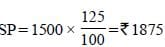Tax paid = ₹75
So, actual SP = 1875 – 75 = ₹1800
Net profit =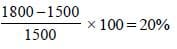SSC CGL Previous Year Questions: Profit & Loss - 3 - Question 2

### Successive discounts of 20% and 10% are equivalent to a single discount of :        (SSC CHSL 2nd Sit. 2015)

Detailed Solution for SSC CGL Previous Year Questions: Profit & Loss - 3 - Question 2

Equivalent discount of 2 successive discounts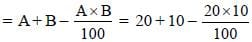= 30 – 2 = 28%

SSC CGL Previous Year Questions: Profit & Loss - 3 - Question 3

### A house was  sold for ₹ y by giving a discount of x%, then the list price was :       (SSC CHSL 1st Sit. 2015)

Detailed Solution for SSC CGL Previous Year Questions: Profit & Loss - 3 - Question 3

Let list price = ₹ z
So, Sale price (y) =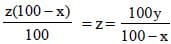SSC CGL Previous Year Questions: Profit & Loss - 3 - Question 4

A fruit seller buys oranges at the rate of ₹ 10 per dozen and sells at the rate of ₹ 12 per dozen. His gain percent is :      (SSC CHSL 1st Sit. 2015)

Detailed Solution for SSC CGL Previous Year Questions: Profit & Loss - 3 - Question 4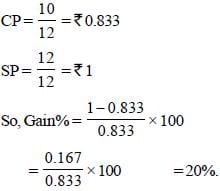SSC CGL Previous Year Questions: Profit & Loss - 3 - Question 5

By selling an article for ₹450. I lose 20%. For what amount, should I sell it to gain 20%?     (SSC CHSL 1st Sit. 2015)

Detailed Solution for SSC CGL Previous Year Questions: Profit & Loss - 3 - Question 5

SP = ₹ 450
Loss = 20%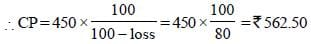SP for getting 20% gain =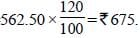SSC CGL Previous Year Questions: Profit & Loss - 3 - Question 6

A fruit seller buys 240 apples for ₹ 600. Some of these apples are bad and are thrown away. He sells the remaining apples at ₹ 3.50 each and makes a profit of ₹ 198. The % of apples thrown away are :          (SSC Sub. Ins. 2015)

Detailed Solution for SSC CGL Previous Year Questions: Profit & Loss - 3 - Question 6

C.P = ₹ 600
Let number of apples thrown = x
So number of apples left = 240 – x
So S.P. = (240 – x) 3.50
= 840 – 3.5x
So 840 – 3.5 x – 600 = 198
240 – 3.5 x = 198
x = 42/3.5 = 12
So % age of apples thrown = (12/240) x 100 = 5%

SSC CGL Previous Year Questions: Profit & Loss - 3 - Question 7

A man bought a watch at 25% discount on the original price. He got ₹40 more than the original price by selling it at 140% of the price at which he bought. The price of buying the watch was:  (SSC Sub. Ins. 2015)

Detailed Solution for SSC CGL Previous Year Questions: Profit & Loss - 3 - Question 7

CP = 0.75 × MP
SP = MP + 40
1.4 CP = M.P + 40
1.4 (0.75) MP = M.P + 40
1.05 MP = M.P. + 40
(1.05 – 1) MP = 40
M.P. = 40/0.05 = ₹800
∴ CP = 0.75 M.P. = 0.75 × 800 = ₹ 600

SSC CGL Previous Year Questions: Profit & Loss - 3 - Question 8

If a shopkeeper purchases cashewnut at ₹250 per kg and sells it at ₹ 10 per 50 grams, then he will have:      (SSC Sub. Ins. 2015)

Detailed Solution for SSC CGL Previous Year Questions: Profit & Loss - 3 - Question 8

C.P. = ₹ 250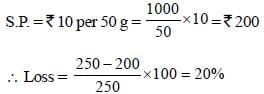SSC CGL Previous Year Questions: Profit & Loss - 3 - Question 9

A trader marks his goods 20% above C.P. but allows his customers a discount  of 10. The C.P. of a blackboard, which is sold for ₹ 216, is:   (SSC Sub. Ins. 2015)

Detailed Solution for SSC CGL Previous Year Questions: Profit & Loss - 3 - Question 9

Marked price = 20 % above CP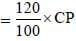Discount = 10%
∴ S.P.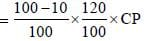= 0.9 × 1.2 × CP = 1.08 CP
S.P. = ₹ 216
C.P.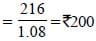SSC CGL Previous Year Questions: Profit & Loss - 3 - Question 10

A sold an article to B at 20% profit and B sold it to C at 15% loss. If A sold it to C at the selling price of B, then A would make       (SSC CGL 1st Sit. 2014)

Detailed Solution for SSC CGL Previous Year Questions: Profit & Loss - 3 - Question 10

Let ₹ 100 be the cost price for A.
S.P. for A = 100 + 20% of 100 = 120
S.P. for B = 120 – 15% of 120 = 102
Profit % =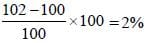SSC CGL Previous Year Questions: Profit & Loss - 3 - Question 11

Ram bought a T.V. with 20% discount on the labelled price. Had he bought it with 30% discount he would have saved ₹ 800. The value of the T.V. set that he bought is       (SSC CGL 1st Sit. 2014)

Detailed Solution for SSC CGL Previous Year Questions: Profit & Loss - 3 - Question 11

Let labelled price of T.V. be ₹ x
Price after 20% discount,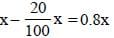Price after 30% discount,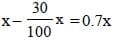According to question
0.8x – 0.7x = 800
x = 800 × 10 = 8000

SSC CGL Previous Year Questions: Profit & Loss - 3 - Question 12

A dealer of scientific instruments allows 20% discount on the marked price of the instruments and still makes a profit of 25%. If his gain over the sale of an instrument is ₹ 150, find the marked price of the instrument.      (SSC CGL 1st Sit. 2014)

Detailed Solution for SSC CGL Previous Year Questions: Profit & Loss - 3 - Question 12

Let marked price of the instrument be ₹ x
Selling price, S.P. = x – (20/100)x = 0.8x
Cost price, C.P. = C.P. + (25/100)C.P. = 0.8x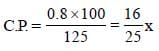x = 25/16 C.P.
Given that 25/100 C.P = 150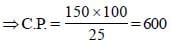Marked price x =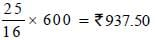SSC CGL Previous Year Questions: Profit & Loss - 3 - Question 13

A shopkeeper allows 10% discount on goods when he sells without credit. Cost price of his goods is 80% of his selling price. If he sells his goods by cash, then his profit is       (SSC CGL 1st Sit. 2014)

Detailed Solution for SSC CGL Previous Year Questions: Profit & Loss - 3 - Question 13

Let marked price of goods be ₹ 100.
Selling price of goods = 100 - (10/100) x 100 = ₹90
Cost price of goods is 80% of its selling price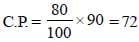Profit on goods = (90 – 72) = ₹ 18
Profit % = (18/72) x 100 = 25%.

SSC CGL Previous Year Questions: Profit & Loss - 3 - Question 14

A man sold his watch at a loss of 5%. Had he sold it for ₹ 56.25 more, he would have gained 10%. What is the cost price of the watch (in ₹) ? (SSC CHSL 2014)

Detailed Solution for SSC CGL Previous Year Questions: Profit & Loss - 3 - Question 14

Let Cost Price of watch be ₹ x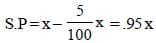If S.P = 0.95x + 56.25 then profit = 10%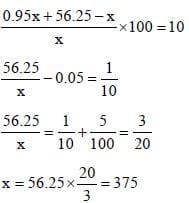SSC CGL Previous Year Questions: Profit & Loss - 3 - Question 15

The marked price of an item is twice the cost price. For a gain of 15%, the discount should be        (SSC CHSL 2014)

Detailed Solution for SSC CGL Previous Year Questions: Profit & Loss - 3 - Question 15

Marked Price, M = 2C, where C is cost price for 15% gain, S.P. = C + (15/100)C = 1.15C
Let discount be x%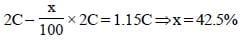SSC CGL Previous Year Questions: Profit & Loss - 3 - Question 16

The marked price of a saree is ₹ 200. After allowing a discount of 20% on the marked price, the shopkeeper makes a profit of ₹ 16. Find the gain percent.      (SSC CHSL 2014)

Detailed Solution for SSC CGL Previous Year Questions: Profit & Loss - 3 - Question 16

Selling price = Marked price – Discount
= 200 – 20% of 200 = 160
Cost Price = 160 – 16 = 144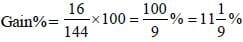SSC CGL Previous Year Questions: Profit & Loss - 3 - Question 17

A retailer buys a sewing machine at a discount of 15% and sells it for ₹1955. Thus he makes a profit of 15%. The discount is         (SSC Sub. Ins. 2014)

Detailed Solution for SSC CGL Previous Year Questions: Profit & Loss - 3 - Question 17

Let original price of sewing machine be ₹ x
Retailer bought it at x - (15/100)x = 0.85x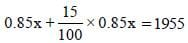⇒ 85x + 12.75x = 1955 × 100
⇒ 97.75x = 195500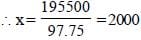Discount is (15/100) x 2000 = ₹ 300

SSC CGL Previous Year Questions: Profit & Loss - 3 - Question 18

Mahesh earned a profit of 20% by selling 60 apples at the rate of  ₹ 42.50 for 5 apples. Then the total cost, at which the apples were bought is (SSC Sub. Ins. 2014)

Detailed Solution for SSC CGL Previous Year Questions: Profit & Loss - 3 - Question 18

Selling price of 5 apples = ₹42.50
Selling price of 60 apples = (42.5/5)x60 = 510
C.P + Profit = S.P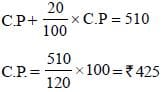SSC CGL Previous Year Questions: Profit & Loss - 3 - Question 19

A tea-merchant professes to sell tea at cost price but uses a false weight of 900 gram for a  kilogram. The profit percent in his transaction is       (SSC Sub. Ins. 2014)

Detailed Solution for SSC CGL Previous Year Questions: Profit & Loss - 3 - Question 19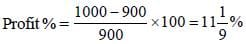SSC CGL Previous Year Questions: Profit & Loss - 3 - Question 20

A shopkeeper sold an item for ₹ 1,800 at a discount of 10% and gained ₹ 200. Had he not given the discount, his gain would be       (SSC Multitasking 2014)

Detailed Solution for SSC CGL Previous Year Questions: Profit & Loss - 3 - Question 20

Let M be the marked price.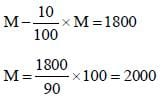Cost Price, C = 1800 – 200 = 1600
If no discount is given Profit ₹ 2000 – ₹ 1600 = ₹ 400

SSC CGL Previous Year Questions: Profit & Loss - 3 - Question 21

Rita purchased a car with a marked price of ₹ 2,10,000 at a discount of 5%. If the sales tax charged is 10%, find the amount she has to pay.      (SSC Multitasking 2014)

Detailed Solution for SSC CGL Previous Year Questions: Profit & Loss - 3 - Question 21

Selling price of car; S.P. = 2, 10,000 – (5/100) x 2,10,000 = 1,99,500
Sales tax charged is 10%
Total cost for Rita = 1,99,500 + (10/100) x 1,99,500 = ₹ 2,19,450

SSC CGL Previous Year Questions: Profit & Loss - 3 - Question 22

A cycle merchant allows 25% discount on the marked price of the cycles and still makes a profit of 20%. If he gains ₹ 360 over the sale of one cycle, find the marked price of the cycle.       (SSC Multitasking 2014)

Detailed Solution for SSC CGL Previous Year Questions: Profit & Loss - 3 - Question 22

Let M be the market price and C be the cost price of the cycle.
Selling price,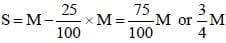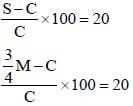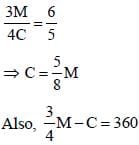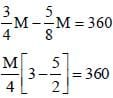M = 360 × 4 × 2 = ₹ 2,880

SSC CGL Previous Year Questions: Profit & Loss - 3 - Question 23

If a person lost 8% by selling an article for ₹ 1,035, he bought the article for      (SSC Multitasking 2014)

Detailed Solution for SSC CGL Previous Year Questions: Profit & Loss - 3 - Question 23

Person bought the article for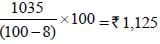SSC CGL Previous Year Questions: Profit & Loss - 3 - Question 24

Aman sells two watches at ₹ 99 each. On one he gets 10% profit and on the other he loses 10%. His net gain or loss percent is       (SSC Multitasking 2014)

Detailed Solution for SSC CGL Previous Year Questions: Profit & Loss - 3 - Question 24

Cost price of watch on which he get 10% Profit,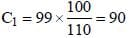Cost Price of watch on which he losses 10%,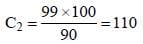Net loss%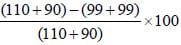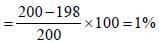SSC CGL Previous Year Questions: Profit & Loss - 3 - Question 25

A bookseller sells a book at a profit of 10%. If he had bought it at 4% less and sold it for ₹ 6 more, he would have gained 18(3/4)%. The cost price of the book is      (SSC Multitasking 2014)

Detailed Solution for SSC CGL Previous Year Questions: Profit & Loss - 3 - Question 25

Let C be the cost price of book
Selling price, S =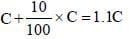If cost price is 6% less, C' =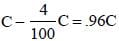S' = 1.1C + 6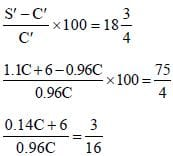14C + 600 = 18C
4C = 600
C = ₹150

SSC CGL Previous Year Questions: Profit & Loss - 3 - Question 26

The price of an article is first decreased by 20% and then increased by 30%. if the resulting price is ₹ 416, the original price of the article is.    (SSC CGL 2nd Sit. 2013)

Detailed Solution for SSC CGL Previous Year Questions: Profit & Loss - 3 - Question 26

If the original price of article be ₹ x, then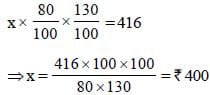SSC CGL Previous Year Questions: Profit & Loss - 3 - Question 27

A tradesmen sold an article at a loss of 20%. if the selling price had been increased by ₹100. there would have been a gain of 5%. The cost price of the article (in ₹) was       (SSC CGL 2nd Sit. 2013)

Detailed Solution for SSC CGL Previous Year Questions: Profit & Loss - 3 - Question 27

C.P. of article = ₹ x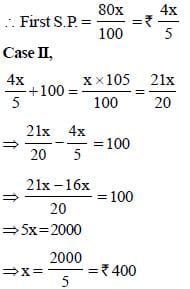SSC CGL Previous Year Questions: Profit & Loss - 3 - Question 28

The list price of an article is ₹160 and a customer buys it for ₹122.40 after two successive discounts. If the first discount is 10%, then second discount is      (SSC CGL 2nd Sit. 2013)

Detailed Solution for SSC CGL Previous Year Questions: Profit & Loss - 3 - Question 28

S.P. after a discount of 10% =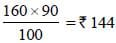Second discount = 144 – 122.40 = ₹ 21.6
If the second discount be x %, then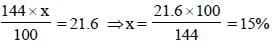SSC CGL Previous Year Questions: Profit & Loss - 3 - Question 29

A shopkeeper earns a profit of 12% on selling a book at 10% discount on printed price. The ratio of the cost price to printed price of the book is       (SSC CGL 2nd Sit. 2013)

Detailed Solution for SSC CGL Previous Year Questions: Profit & Loss - 3 - Question 29

C.P. of the book = ₹ x
Printed price = ₹ y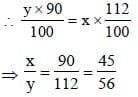SSC CGL Previous Year Questions: Profit & Loss - 3 - Question 30

A shopkeeper marks his goods 20% above his cost price and gives 15% discount on the marked price. His gain percent is       (SSC CGL 2nd Sit. 2013)

Detailed Solution for SSC CGL Previous Year Questions: Profit & Loss - 3 - Question 30

If the C.P. of goods be ₹100, then
Marked price = ₹120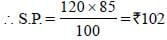∴ Profit percent = 2%.

SSC CGL Previous Year Questions: Profit & Loss - 3 - Question 31

A CD was sold at a profit of 12(1/2)%. If it had been sold at a profit of 15%, it would have gained him ₹ 10 more. The cost prices of CD is (in ₹)      (SSC CGL 1st Sit. 2013)

Detailed Solution for SSC CGL Previous Year Questions: Profit & Loss - 3 - Question 31

Ist case :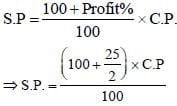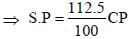...(i)
IInd case :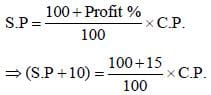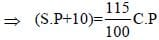...(2)
Dividing equation (1) by (2)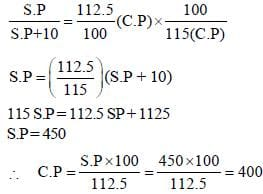SSC CGL Previous Year Questions: Profit & Loss - 3 - Question 32

On the eve of Gandhi Jayanti, Gandhi Ashram declared a 25% discount on silk. If selling price of a silk saree is ₹ 525, what is its marked price ?       (SSC CGL 1st Sit. 2013)

Detailed Solution for SSC CGL Previous Year Questions: Profit & Loss - 3 - Question 32

Let the marked price be ₹ x.
∵ S.P. = (x – 25% of x) = (3/4)x
But, S.P = ₹ 525
∴ (3/4)x = 525 ⇒ x = 700

SSC CGL Previous Year Questions: Profit & Loss - 3 - Question 33

Kabir buys an article with 25% discount on its marked price. He makes a profit of 10% by selling it at ₹ 660. The marked price is       (SSC CGL 1st Sit. 2013)

Detailed Solution for SSC CGL Previous Year Questions: Profit & Loss - 3 - Question 33

Let the marked price be ₹x.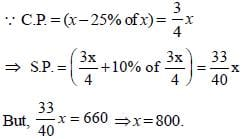SSC CGL Previous Year Questions: Profit & Loss - 3 - Question 34

The marked price of a radio set is ₹480. The shopkeeper allows a discount of 10% and gains 8%. If no discount is allowed, his gain percent would be      (SSC CGL 2nd Sit. 2013)

Detailed Solution for SSC CGL Previous Year Questions: Profit & Loss - 3 - Question 34

If the C.P. of radio be ₹ x, then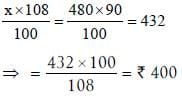If no discount is allowed, Gain = 480 – 400 = ₹ 80
Gain percent = (80/400) x 100 = 20%.

SSC CGL Previous Year Questions: Profit & Loss - 3 - Question 35

The marked price of a table is ₹ 12,000. If it was sold for ₹10,500 after allowing a certain discount, then the rate of discount is       (SSC CGL 2nd Sit. 2013)

Detailed Solution for SSC CGL Previous Year Questions: Profit & Loss - 3 - Question 35

Discount = 12000 – 10500 = ₹ 1500
If the discount per cent be x, then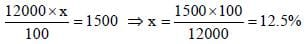SSC CGL Previous Year Questions: Profit & Loss - 3 - Question 36

A dozen pairs of socks quoted at ₹ 180 are available at discount of 20%. How many pairs of socks can be bought for ₹ 48?       (SSC CGL 2nd Sit. 2013)

Detailed Solution for SSC CGL Previous Year Questions: Profit & Loss - 3 - Question 36

∵ S.P. of a dozen pairs of socks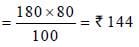∴ S.P. of 1 pair of socks = 144/12 = ₹12
∴ No of pairs available for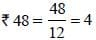SSC CGL Previous Year Questions: Profit & Loss - 3 - Question 37

An article is sold for ₹300 at a profit of 20%. Had it been sold ₹235, the loss percentage would have been      (SSC CGL 2nd Sit. 2013)

Detailed Solution for SSC CGL Previous Year Questions: Profit & Loss - 3 - Question 37

C.P. of the article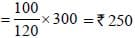On selling at ₹ 235,
Loss percent = (15/250) x 100 = 6%.

SSC CGL Previous Year Questions: Profit & Loss - 3 - Question 38

A fruit seller buys some oranges at the rate of 4 for ₹ 10 and an equal number more at 5 for ₹ 10. He sells the whole lot at 9 for ₹ 20. What is his loss or gain percent?       (SSC CGL 2nd Sit. 2013)

Detailed Solution for SSC CGL Previous Year Questions: Profit & Loss - 3 - Question 38

Let 20 apples of each type be bought.
C.P. of 40 apples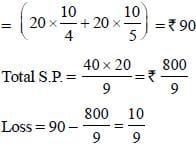∴ Loss percent =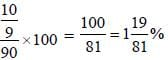SSC CGL Previous Year Questions: Profit & Loss - 3 - Question 39

A reduction in the price of apples enables a person to purchase 3 apples for ₹ 1 instead of ₹ 1.25. What is the % of reduction in price (approximately)?        (SSC CGL 2nd Sit. 2013)

Detailed Solution for SSC CGL Previous Year Questions: Profit & Loss - 3 - Question 39

Percentage decrease = (25/125)x100 = 20%

SSC CGL Previous Year Questions: Profit & Loss - 3 - Question 40

A retailer purchased radiosets at the rate of  ₹ 400 each from a wholesaler. He raised the price by 30% and then allowed a discount of 8% on each set. His profit will be      (SSC CGL 2nd Sit. 2013)

Detailed Solution for SSC CGL Previous Year Questions: Profit & Loss - 3 - Question 40

Marked price of a ratio set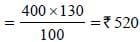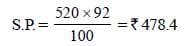∴ Gain percent =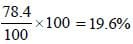## SSC CGL (Tier - 1) - Previous Year Papers (Topic Wise)

250 tests
Information about SSC CGL Previous Year Questions: Profit & Loss - 3 Page
In this test you can find the Exam questions for SSC CGL Previous Year Questions: Profit & Loss - 3 solved & explained in the simplest way possible. Besides giving Questions and answers for SSC CGL Previous Year Questions: Profit & Loss - 3, EduRev gives you an ample number of Online tests for practice

250 tests